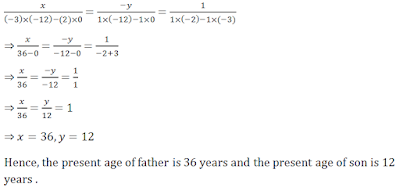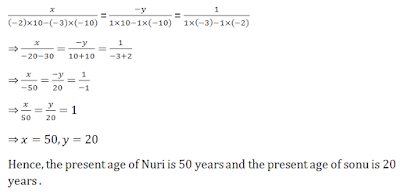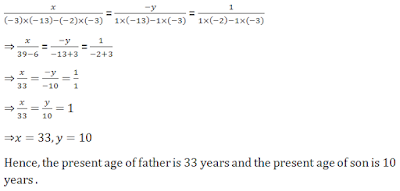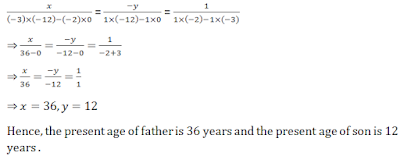## Chapter 3 Pair of Linear Equations in Two Variables R.D. Sharma Solutions for Class 10th Math Exercise 3.9

Exercise 3.9

1A father is three times as old as his son. After twelve years, his age will be twice as that of his son then. Find the their present ages .

Solution

Let the present age of father be x years and the present age of son be y years. Father is three times as old as his son. Thus, we have
x = 3y
⇒ x-3y = 0
After 12 years, father’s age will be (x+12) years and son’s age will be (y+12) years . Thus using the given information, we have
x+12=2(y+12)
⇒ x+12=2y+24
⇒ x-2y-12=0
So, we have two equations
x-3y=0
x-2y-12=0
Here, x and y are unknows . We have to solve the above equations for x and y .
By using cross-multiplication, we have2. Ten years later, A will be twice as old as B and five years ago, A was three times as old as B. What are the present ages of A and B ?

Solution

Let the present age of A be x years and the present age of B be y years.
After 10 years, A’s age will be (x+10) years and B’s age will be (y+10) years. Thus using the given information, we have
x+10=2(y+10)
⇒ x+10 = 2y+20
⇒ x – 2y – 10 = 0
Before 5 years, the age of A was (x-5) years and the age of B was (y-5) years . Thus using the given information, we have
x-5=3(y-5)
⇒ x – 5 = 3y – 15
⇒ x – 3y + 10 =0
So, we have two equations
x-2y-10=0
x-3y+10=0
Here, x and y are unknowns. We have to solve the above equations for x and y .
By using cross – multiplication, we have3. Five years ago, Nuri was thrice as old as Sonu. Ten years later, Nuri will be twice as old as Sonu. How old are Nuri and Sonu ?

Solution

Let the present age of Nuri be x years and the present age of Sonu be y years. After 10 years, Nuri’s age will be(x + 10) years and the age of Sonu will be(y + 10) years. Thus using the given information, we have
x + 10 = 2(y+10)
⇒ x + 10 = 2y + 20
⇒ x – 2y – 10 = 0
Before 5 years, the age of Nuri was(x – 5)years and the age of Sonu was(y – 5)years. Thus using the given information, we have
x - 5 = 3 (y-5)
⇒ x - 5 = 3y – 15
⇒ x – 3y + 10 = 0
So, we have two equations
x - 2y -10 = 0
x - 3y + 10 = 0
Here, x and y are unknowns. We have to solve the above equations for x and y. By using cross-multiplication, we have4. Six years hence a man's age will be three times the age of his son and three years ago he was nine times as old as his son. Find their present ages.

Solution

Let the present age of the man be x years and the present age of his son be y years. After 6 years, the man’s age will be (x+6) years and son’s age will be (y+6) years . Thus using the given information, we have
x + 6 = 3(y+6)
⇒ x + 6 = 3y + 18
⇒ x – 3y – 12 = 0
Before 3 years, the age of the man was (x-3) years and the age of son’s was (y-3) years. Thus using the given information, we have
x – 3 = 9(y-3)
⇒ x -3 = 9y – 27
⇒ x – 9y + 24 = 0
So, we have two equations
x - 3y – 12 = 0
x - 9y + 24 = 0
Here, x and y are unknowns. We have to solve the above equations for x and y. By using cross-multiplication, we have5. The present age of a father is three years more than three times the age of the son. Three years hence father's age will be 10 years more than twice the age of the son. Determine their present ages .

Solution

Let the present age of father be x years and the present age of his son be y years. After 10 years, father’s age will be (x+10) years and son’s age will be (y+10) years . Thus using the given information, we have
x + 10 = 2(y+10)
⇒ x + 10 = 2y + 20
⇒ x – 2y – 10 = 0
Before 10 years, the age of father was (x-10)years and the age of son was (y-10) years . Thus using the given information, we have
x – 10 = 12(y-10)
⇒ x – 10 = 12y – 120
⇒ x – 12y + 110 = 0
Here, x and y are unknowns. We have to solve the above equations for x and y. By using cross-multiplication, we have6. The present age of a father is three years more than three times the age of the son. Three years hence father's age will be 10 years more than twice the age of the son. Determine their present ages.

Solution

Let the present age of father be x years and the present age of his son be y years. The present age of father is three years more than three times the age of the son. Thus, we have
x = 3y + 3
⇒ x – 3y – 3 = 0
After 3 years, father’s age will be (x+3) years and son’s age will be (y+3) years.
Thus using the given information, we have
x + 3 = 2(y+3) + 10
⇒ x + 3 = 2y + 6 + 10
⇒ x – 2y -13 = 0
So, we have two equations
x – 3y - 3 = 0
x - 2y - 13 = 0
Here, x and y are unknowns. We have to solve the above equations for x and y. By using cross-multiplication, we have7. A father is three times as old as his son. In 12 years time, he will be twice as old as his son. Find the present ages of father and the son .

Solution

Let the present age of father be x years and the present age of his son be y years. The present age of father is three times the age of the son. Thus, we have
x = 3y
⇒ x – 3y = 0
After 12 years , father’s age will be (x+12) years and son’s age will be (y+12) years . Thus using the given information , we have
x + 12 = 2(y+2)
⇒ x + 12 = 2y + 24
⇒ x – 2y – 12 = 0
Here, x and y are unknowns. We have to solve the above equations for x and y. By using cross-multiplication, we have8. Father's age is three times the sum of age of his two children. After 5 years his age will be twice the sum of ages of two children. Find the age of father.

Solution

Let the present age of father be x years and the present ages of his two children’s be y and z years.
The present age of father is three times the sum of the ages of the two children’s. Thus, we have
x = 3(y+z)
⇒ y+z = x/3
After 5 years, father’s age will be (x+5) years and the children’s age will be (y+5) and (z+5) years .
Thus using the given information,we have
x + 5 = 2{(y+5) + (z+5)}
⇒ x + 5 = 2(y+5+z+5)
⇒ x = 2(y+z) + 20 – 5
⇒ x = 2(y+z) + 15
So, we have two equations
y+z = x/3
x = 2(y+z) + 15
Here x,y and z are unknowns . We have to find the value of x .
Substituting the value of (y+z) from the first equation in the second equation, we have
By using cross – multiplication , we have9. Two years ago, a father was five times as old as his son. Two year later, his age will be 8 more than three times the age of the son. Find the present ages of father and son.

Solution

Let the present age of father be x years and the present age of his son be y years. After 2 years, father’s age will be (x+2) years and the age of son will be (y+2) years. Thus using the given information, we have
x+2 = 3(y+2)+8
⇒ x+2=3y+6+8
⇒ x-3y-12=0
Before 2 years, the age of father was (x-2) years and the age of son was (y-2) years . Thus using the given information , we have
x-2 =5(y-2)
⇒ x – 2 = 5y – 10
⇒ x – 5y + 8 = 0
So, we have two equations
x – 3y – 12 = 0
x – 5y + 8 = 0
Here, x and y are unknowns . We have to solve the above equations for x and y .
By using cross – multiplication, we have10. A is elder to B by 2 years. A's father F is twice as old as A and B is twice as old as his sister S. If the ages of the father and sister differ by 40 years, find the age of A.

Solution

Let the present ages of A, B, F and S be x, y, z and t years respectively.
A is elder to B by 2 years. Thus, we have x = y + 2
F is twice as old as A. Thus, we have z = 2x
B is twice as old as S. Thus, we have y = 2t
The ages of F and S is differing by 40 years. Thus, we have z – t = 40
So, we have four equations
x = y+2 …..(1)
z = 2x, ……(2)
y = 2t, ……(3)
z – t = 40 …(4)
Here x, y, z and t are unknowns. We have to find the value of x.
By using the third equation, the first equation becomes x = 2t + 2
From the fourth equation, we have t = z – 40
Hence, we have
x = 2(z – 40) + 2
= 2z – 80 + 2
= 2z – 78
Using the second equation, we have
x = 2 × 2x – 78
⇒ x = 4x – 78
⇒ 4x – x = 78
⇒ 3x = 78
⇒ x = 78/3
⇒ x = 26
Hence , the age of A is 26 years .

11. The ages of two friends Ani and Biju differ by 3 years. Ani's father Dharma is twice as old as Ani and Biju as twice as old as his sister Cathy. The ages of Cathy and Dharam differ by 30 years. Find the ages of Ani and Biju.

Solution

Let the present ages of Ani, Biju, Dharam and Cathy be x, y, z and t years respectively.
The ages of Ani and Biju differ by 3 years. Thus, we have
x – y = ± 3
⇒ x = y ± 3
Dharam is twice as old as Ani. Thus, we have z = 2x
Biju is twice as old as Cathy. Thus, we have y = 2t
The ages of Cathy and Dharam differ by 30 years. Clearly, Dharam is older than Cathy. Thus, we have z-t = 30
So, we have two systems of simultaneous equations

(i) x = y + 3,
z = 2x,
y = 2t,
z-t = 30

(ii) x = y – 3 ,
z = 2x,
y = 2t,
z-t = 30
Here x, y, z and t are unknowns. We have to find the value of x and y.

(i) By using the third equation, the first equation becomes x = 2t + 3
From the fourth equation, we have
t = z -30
Hence, we have
x = 2(z-30)+3
= 2z – 60 + 3
= 2z – 57
Using the second equation, we have
x = 2 × 2x – 57
⇒ x = 4x – 57
⇒ 4x – x = 57
⇒ 3x = 57
⇒ x = 57/3
⇒ x = 19
From the first equation, we have
x = y+3
⇒ y = x – 3
⇒ y = 19 – 3
⇒ y = 16
Hence, the age of Ani is 19 years and the age of Biju is 16 years.

(ii) By using the third equation becomes x = 2t – 3
From the fourth equation, we have
t = z – 30
Hence, we have
x = 2(z-30) – 3
= 2z – 60 – 3
= 2z – 63
Using the second equation, we have
x = 2× 2x – 63
⇒ x = 4x – 63
⇒ 4x – x = 63
⇒ 3x = 63
⇒ x = 63/3
⇒ x = 21
From the first equation, we have
x = y-3
⇒ y = x + 3
⇒ y = 21+3
⇒ y = 24
Hence, the age of Ani is 21 years and the age of Biju is 24 years .
Note that there are two possibilities .

12. Two years ago , Salim was thrice as old as his daughter and six years later, he will be four years older than thrice her age . How old are they now ?

Solution

Let the present ages of Salim be x years and that of her daughter be y years.
Two years ago, the age of Salim was (x - 2) years and that of her daughter was (y -2).
It is given that Salim was thrice as old as her daughter two years ago. So,
x - 2 = 3(y - 2)
⇒ x - 2 = 3y - 6
⇒ x - 3y = -4 .....(i)
Six years later, the age of Salim will be (x + 6) and that of her daughter will be (y + 6).
∴ x + 6 = 2(y + 6) + 4
⇒ x - 2y = 10 .....(ii)
Subtracting (ii) from (i), we get
-y = -14
⇒ y = 14
Putting y = 14 in (ii), we get
x - 28 = 10
⇒ x = 38
Hence, the present age of Salim is 38 years and that of her daughter is 14 year .

13. The age of the father is twice the sum of the age of his two children . After 20 years, his age will be equal to the sum of the ages of his children . Find the age of the father .

Solution

Let the present age of the father be x years and the sum of the present ages of his two children be y years.
Now according to the given conditions,

Case I: x = 2y
⇒ x - 2y = 0 .....(i)

Case II: After 20 years, the age of the father will be (x + 20) years and the sum of the ages of the two children will be y + 20 + 20 = (y + 40) years.
So, x + 20 = y + 40
⇒ x - y = 20 .....(ii)
Subtracting (ii) from (i), we get
- y = -20
⇒ y = 20
Putting y = 20 in (i), we get
x - 40 = 0
⇒ x = 40
Hence, the present age of the father is 40 years.